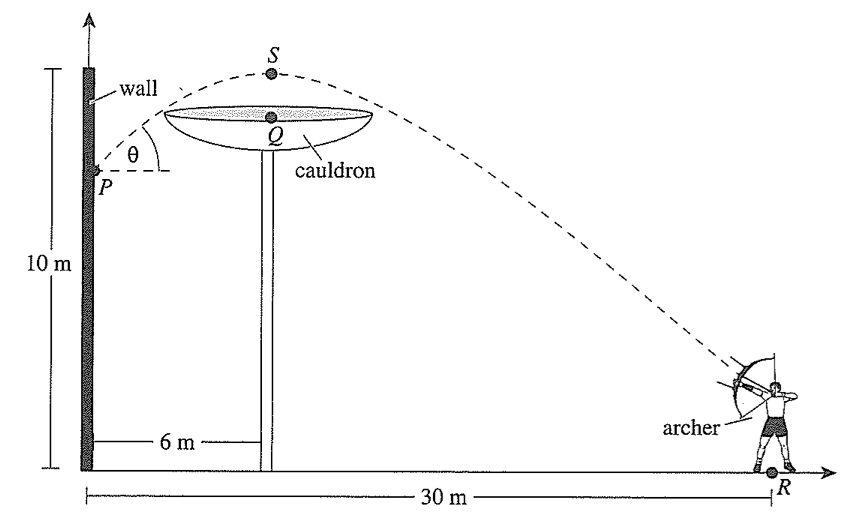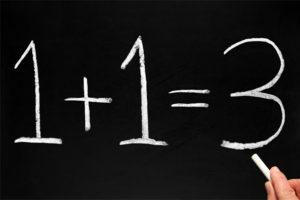Posted on

## Significant Figures and Scientific NotationFor many students, the concepts of significant figures and scientific notation are initially daunting and confusing. It is not unusual for a student to confuse the idea of rounding to a certain number of decimal places as being the same as rounding to a certain number of significant figures. Adding the concept of scientific notation into the mix can lead to the student being so uncertain as to how to write their answer for a given question.

Posted on

## A “Problematic” ArcherStudents often bring in questions that are taken or adapted from sources outside of the usual textbooks. This is especially true with regards to revision material when leading up to topic test or other major assessment. One question of this type that really caught my attention was from a "Smart Study" guide given to students preparing for a SAC (i.e. major test) on Functions and Graphs. The diagram is shown here:(note this diagram remains the intellectual property of the copyright holder)

Posted on

## Statistics and the “Scientific Method”# The “Scientific Method”

Most secondary school students are taught about the “Scientific Method” during their general science classes. From these early classes, students are encouraged to believe that all scientists follow an objective and almost linear process in their research. The process is generally described as something like this:

1. an unusual observation is made
2. an explanation is proposed for this observation
3. experiments are performed to test the explanation
4. after repeated experiments a theory is formed
5. experiments are performed to validate the theory
6. once validated the theory becomes a new scientific law
7. the scientific laws are used to predict new observations
Posted on

## Statistics in “APA Format”Mathematics is an ancient discipline, developed through many cultures and with many different ways of representing numbers and operations. Fortunately, there is a accepted convention that now governs how mathematics is represented around the world and it is through this convention that many mathematicians are able to exchange ideas independent of whether they speak the same (non-mathematical) language. It is not surprising, therefore, that statistics (a branch of mathematics) also has a convention on how statistics are represented.

## Statistical Conventions

In the statistics discipline we are typically dealing with one of two groups, the population or the sample. The population we are working with should be denoted by a capital Roman letter (e.g.$X$ ) and an individual from that population should be denoted with a lower case Roman letter (e.g.$x$ ). When we take a sample from the population, we are getting a set of$x$ ‘s out of our population$X$  (i.e each$x \in X$ )

Posted on

## Infallible Calculator?You may have seen the claim on the Internet that millions of people get the answer to the equation$6 \div 2\left(1 + 2\right)$  (or similar) wrong. You may have even read the explanations by some people about why the answer should be 9 or should be 1. Let me clear it up and explain why the answer is most definitely 1, but why the calculator (and others) give 9.

Firstly, the important thing to recognise is that this equation, like all others, is subject to the Order of Operations, which states that we must solve the contents of the brackets first. Thus our equation becomes$6 \div 2(3)$ .

Posted on

## A “Novel” approach to polynomial division…During the last few weeks I have been working with some Year 11 students from a local private school that has a fairly good reputation. These students have been working, in particular, on cubic and quartic functions, including the use of polynomial division. The students in question are taught by two different teachers at the school, including their school’s Head of Mathematics, a highly experienced and long serving teacher by all accounts. All of these students are certainly above average students, but like most of their peers, have had little exposure to the long division method in primary school, mostly due to the prevalence of handheld calculators.

Whilst working with these students, each of them has independently demonstrated to me a short-cut method taught to them at school for polynomial division. This short-cut method is well known and quite useful when applied correctly. Unfortunately, when originally demonstrated to the students (judging by each of their theory books), their respective teachers each made the same monumental error and taught the students a technique that would work only in certain circumstances. The following are some of the examples given to these students in class:

Posted on

## Proof that 1 + 1 = 1. Not 2!?!Here is a quick demonstration of what can go wrong when you violate the rules of mathematics.

1. Let$a = 1$  and$b = 1$ .

Posted on

## Lies, Damned Lies and (Misused) Statistics…Mark Twain is credited with stating that there are three kinds of lies, "Lies, damned lies and statistics". As a statistician, I often hear people quoting this to me to which I often point out that Twain was only partially correct. There are three kinds of lies, "Lies, damned lies and lies told by misusing statistics". It appears that once again in Australia, vital funding choices are about to be made through the use of a faulty statistic, the citation count.

During this past week I’ve been happily working away with my students, teaching them all I can about the wonderful world of mathematics and statistics. In particular, we’ve looked at what inferences you can draw from a set of descriptive statistics. One particular example in their textbooks asks the students to compare the pulse rates of 21 adult males and 22 adult females and make an inference about male and female pulse rates. As expected from the data supplied, these students conclude that females, in general, have higher and more variable pulse rates than the males. Whilst that is a correct conclusion from the supplied data, I always ask the students if there is anything they might be concerned about with regards to this data and how it was collected.